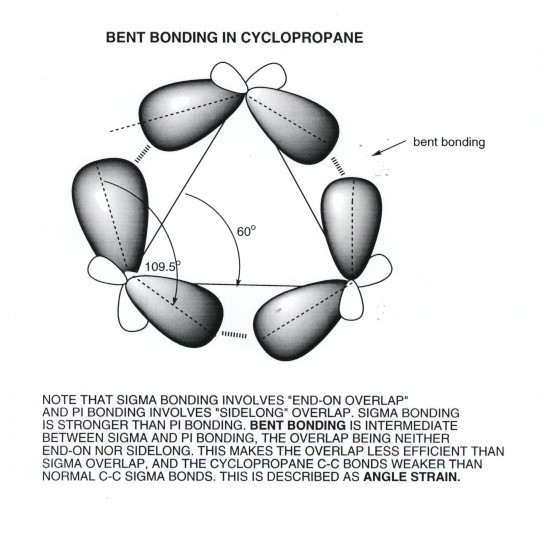TOP

# Ring Strain in Cyclopropane

## The Large Ring Strain in Cyclopropane

• Unlike acyclic hydrocarbons, cyclic hydrocarbons may have strain which is inherent in their cyclic structures. This is called RING STRAIN.

• Ring Strain consists primarily of two diffferent kinds of strain: (1)torsional strain and (2)angle strain.Torsional strain, of course, arises when bonds are not ideally staggered. In most ring systems, it is impossible to perfectly stagger all bonds. In cyclohexane, fortuitously, perfect bond staggering occurs in the chair conformation, but no conformation in any other ring allows for such perfect staggering.

• ANGLE STRAIN arises when the C-C-C bonds of the ring depart (because of geometric necessity) from the ideal tetrahedral angle preferred for sp3 carbon. Again, in chair cyclohexane this angle just happens to be virtually identical to the tetrahedral angle, so that neither angle nor torsional strain occurs in cyclohexane in the chair form. Therefore the total ring strain is nil for cyclohexane.

• Cyclopropane, the smallest cycloalkane, is rather highly strained (although it is still easily isolated and stored). The estimated total ring strain in cyclopropane is 28 kcal/mol (from heats of combustion measurements). When this value is compared with the strength of a typical C-C bond (ca. 88 kcal/mol), it can be seen that ring strain substantially weakens the C-C bonds of the ring. Hence, cyclopropane is much more reactive than acyclic alkanes and other cycloalkanes such as cyclohexane and cyclopentane.

• The carbon framework of cyclopropane is planar, by definition.That is , it could not be otherwise, because it takes three points to define a plane, and the positions of the three carbon nuclei define these points. Thus, there is essentially no conformational analysis required for cyclopropane. You might recall that we had earlier pointed out the strain in planar cyclohexane is large, because all of the C-C bonds are eclipsed when the carbon framework is planar. This is also true in cyclopropane, or in any other planar conformation of a cycloalkane. Thus, planar conformations are usually disfavored, but no other conformation is available to cyclopropane. Since there are three C-C bonds in cyclopropane, and each of them is staggered, we can estimate that there is at least 3X3 = 9 kcal/mol of torsional strain in cyclopropane.

• Since the total ring strain in cyclopropane is about 28 kcal/mol, it is evident that the major part of the ring strain must arise from another factor. This is angle strain. Angle strain in cyclopropane arises from the circumstance that the C-C-C angle is forced to be 60 degrees (that of an equilateral triangle), about 49 degrees less than the ideal angle for the strongest C-C bond (the strain angle is ca. 49 degrees.

• Looking at this in a little more detail, we can see that the C-C bonds of cyclopropane are "bent" and not pure sigma bonds. Recall that overlap is greatest when orbitals overlap "end-on", i.e., via sigma bonding. Pi bonds overlap laterally. The overlap in cyclopropane is neither end-on or lateral, but in between. So it is intermediate between sigma and pi bonding. These bonds are also sometimes called "banana bonds".

• The cause of the bent overlap is that the internuclear angle ( the angle between the bond lines connecting the nuclei) is forced to be much smaller (60 degrees) than the interorbital angle (the angle between the axis of the two AO's on a given carbon), which is 109.5 degrees.